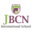Trending ▼   ResFinder# ICSE Class X Prelims 2016 : Mathematics (St. Francis School (SFS), Borivali, Mumbai)

2 pages, 32 questions, 0 questions with responses, 0 total responses,20Yusufi Kapasi JBCN International School, Mumbai
+Fave Message
 Home > yakapasi >   F Also featured on: School PageFormatting page ...

A1 ST. FRANCIS SCHOOL, MT. POINSUR, BORIVALI (W), MUMBAI Sub: MATHEMATICS 1st ASSESSMENT EXAM 2016-17 Marks: 80 10 Class: X Time: 2 hrs Date: /08 /2016. _____________________________________________________________________________________________________ SECTION 1 Question 1 a) Divide 36,465 between A and B so that when their shares are lent out at 10% compound interest per year, the amount that A receives in 7 yrs is the same as what B receives in 5 years. (3 m) b) Solve the given inequation and graph the solution on the number line. (3 m) 2y 3 < y + 1 4y + 7, y R. c) The price of a TV set inclusive of sales tax of 9% is 13407. Find its marked price. If the sales tax is increased to 13%, how much more does the customer pay for the TV? (4 m) Question 2 a) If 8a 5b = 8a + 5b , prove that a = c. (3 m) 8c 5d 8c + 5d b d b) Using factor theorem, show that (x + 4) is a factor of (2x3 + 9x2 + x 12). Hence factorise the given expression completely. (3 m) c) The ages of A and B are in the ratio 7:8. Six years ago, their ages were in the ratio 5:6. Find their present ages. (4 m) Question 3 a) The model of a building is constructed with scale factor 1:30. (i) If the height of the model is 80 cm, find the actual height of the building in metres. (ii) If the actual volume of the tank on the top of the building is 27 m3, find the volume of the tank on the top of the model. (3 m) b) In the figure, PB and QA are perpendicular to the line segment AB. If PO = 6 cm, QO = 9 cm and the area of POB = 120 cm2, find the area of QOA. (3 m) P A O B Q 3 2 1 4 (4 m) 2x 1 c) Find x and y if + 2 4 5 = 4 2 y . Question 4 a) In the given figure, AB is a diameter of a circle with centre O and chord ED is parallel to AB and EAB = 650. Calculate: (i) EBA (ii) BED (iii) BCD. (3 m) E D C A B b) In the given figure, PQ and PR are two equal chords of a circle. Show that the tangent at P is parallel to QR. Q S R T P c) Show that equal chords of a circle subtend equal angles at the centre of the circle. (4 m) SECTION 2 Question 5 P1 Std 10 Mathematics 2015-16 (3 m) Page 1 of 2Formatting page ...